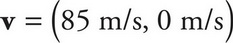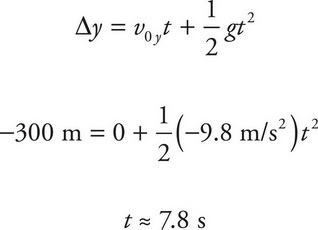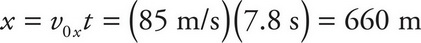# AP Physics 1 Question 378: Answer and Explanation

### Test Information

Question: 378

6. An airtanker is being used to fight a forest fire. It is flying with a ground speed of 85 m/s and maintaining an altitude of 300 m. If it is flying directly towards the fire, at what approximate horizontal distance from the fire should its tanks be open in order for the water dropped to land on the fire? (Assume that the fire is relatively localized.)

• A. 230 m
• B. 250 m
• C. 660 m
• D. 1,700 m

The horizontal distance that the water travels from its drop point is given by x = v0xt. Since the water is dropped from the plane, its initial velocity will be the same as that of the airtanker,. Thus, v0x = 85 m/s. The time it will travel is equivalent to the water's flight time, which can be calculated using the y-direction:Therefore,.